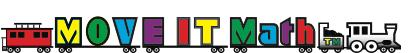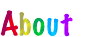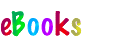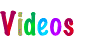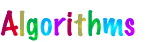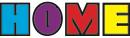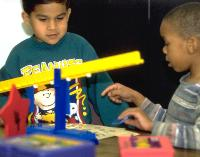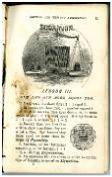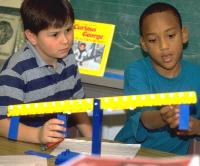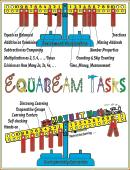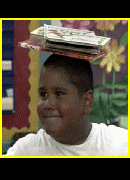Sister: “How are you doing in school?

Brother: “Not good. Too many eagles.

Sister: “Eagles?

Brother: “Yes. One plus one eagles two, two plus two eagles four, three plus three eagles six ...

The equal sign confuses many children. To realize the extent of the confusion, have a class of elementary school children answer true or false for the following number sentences:

5 = 2 + 3, true or false?

7 = 7, true or false?

4 + 6 = 8 + 2, true or false?

You may be amazed at the results. We were, in asking these questions of more than 20,000 grade 1 through grade 8 children. To view the results we obtained, click .

To learn how the children were thinking when they answered the questions, we asked a sample of them to “correct” the ones they had answered false. For 5=2+3, the common “correction” was to rewrite it as 2+3=5 (“because the answer has to be written after the problem”). For 7=7, it was to rewrite it as 7+0=7 or 7x1=7 (“because there has to be a problem”). And for 4+6=8+2, it was to rewrite it as 4+6=10 and 8+2=10 (“because there are two problems”). Summing up, these children thought that the equal sign meant write the answer to a problem to the right of the equal sign.

The results for 7=7 were particularly worrisome. More than half of the grade 1, grade 2, and grade 3 children tested claimed that 7 = 7 was false. For these children, difficulty with fractions was a certainty unless the equal sign in this instance is understood correcly. Consider 1/2+1/4. To add the fractions, one must know that 1/2=2/4, but 1/2=2/4 is of the same form as 7=7, so children who think 7=7 is false will be puzzled by the assertion that 1/2=2/4 is “true” and will be forced to memorize their way through fractions instead of understanding them and thinking their way through them. Learning math through understanding is not only easier but takes one further in the subject.

Equally worrisome was the preponderance of false responses to 4+6=8+2. About 50-60 percent of the grade 1 through grade 5 children tested claimed it was false. Being confused by the equal sign being used to link equivalent quantities portends difficulty with algebra. The following algebraic equations are fundamental to the subject and all are of the form 4+6=8+2.

Associative properties: (a + b) + c = a + (b + c) and (ab)c = a(bc)

Commutative properties: a + b = b + a and ab = ba

Distributive property: a(b + c) = ab + ac

On a calculator, the “equals” key is an operation—a command to “do something.” Pressing it instructs the calculator to “write the answer.” However, in arithmetic, and math in general, “equals” is a relation. It doesn't do anything. It just indicates that what is on one side of the equal sign “is the same as” that which is on the other side. For the three number sentences above, it states that 5 is the same as 2+3, that 7 is the same as itself, and that 4+6 is the same as 8+2.

Thinking of the equal sign on a calculator as meaning “write the answer” is all right, which raises an important point in mathematics: the same symbol in different contexts can mean very different things, just like the same word in different settings can assume very different meanings. (“Wind,” for example, is even pronounced differently if it is used in reference to a breeze versus a baseball pitcher’s “wind up.”) The plus sign in 3+5, for example, means “add,” but in +5, it means “positive” or “to the right of zero” on a number line.

CONTINUED: Next > | 1 | 2 |